# Ncert Maths solutions for Exercise 5.8 class 6 chapter 5 Understanding Elementary Shapes

Ncert Maths Class 6 chapter 5 Understanding Elementary Shapes Exercise 5.8 solutions are given.

Study the textbook lesson Understanding Elementary Shapes very well.

Observe the example problems and solutions given in the textbook.

Observe the given solutons and try them in your own method.

You can see

Ncert maths solutions for class 6
Understanding elementary shapes

Exercise 5.1

Exercise 5.2

Exercise 5.3

Exercise 5.4

Exercise 5.5

Exercise 5.6

Exercise 5.7

Exercise 5.8

Exercise 5.9

M

# Exercise 5.8 chapter 5 solutions class 6 Ncert Maths

Chapter 5

Understandind Elementary Shapes

Exercise 5.8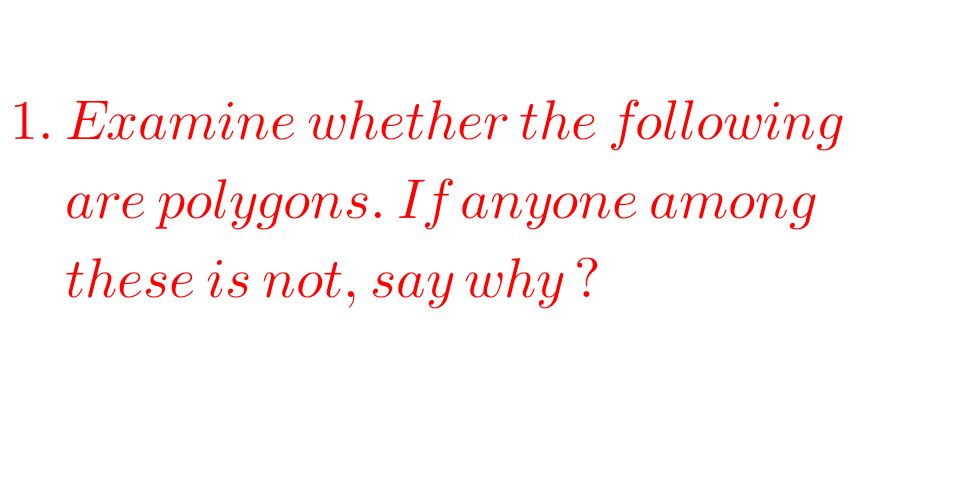F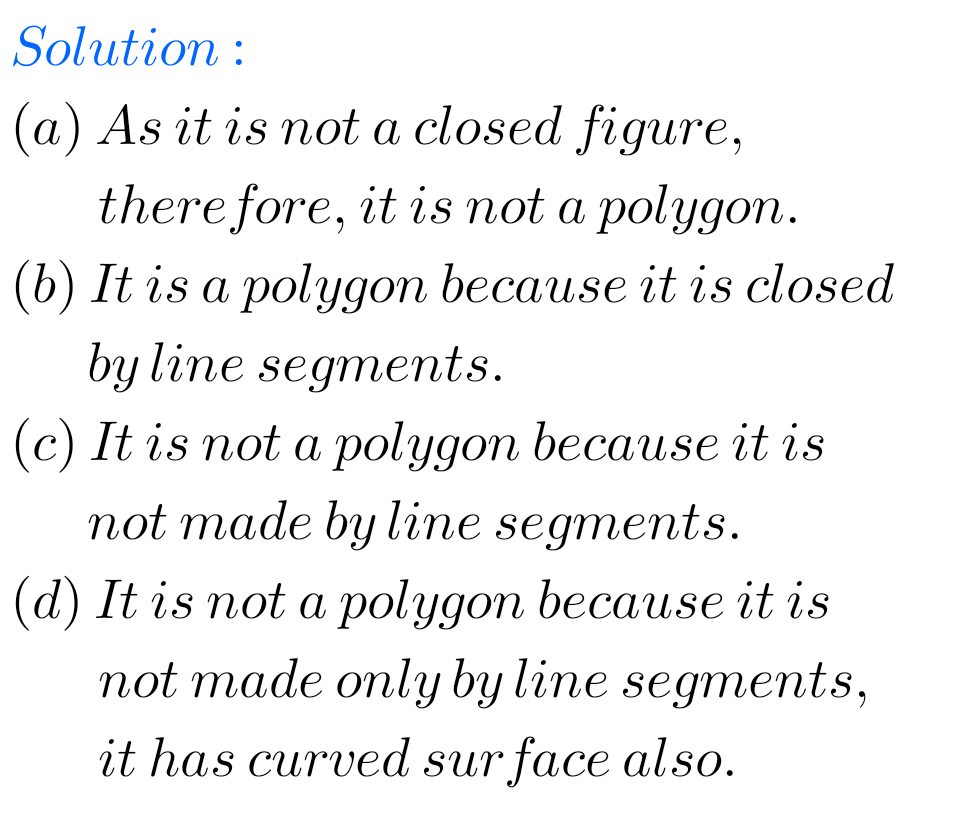F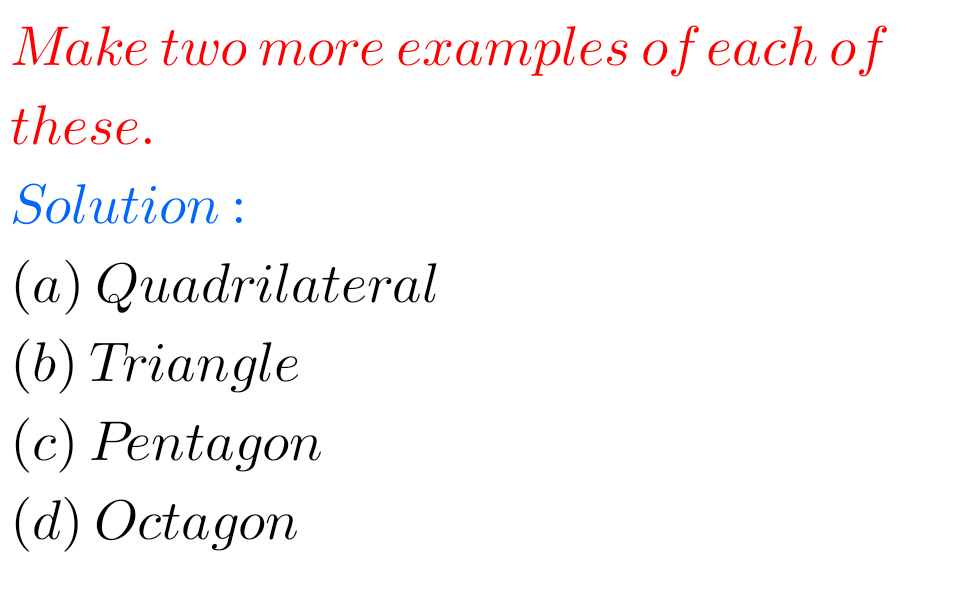## Maths solutions Ncert Class 6 maths Exercise 5.8 Understanding Elementary Shapes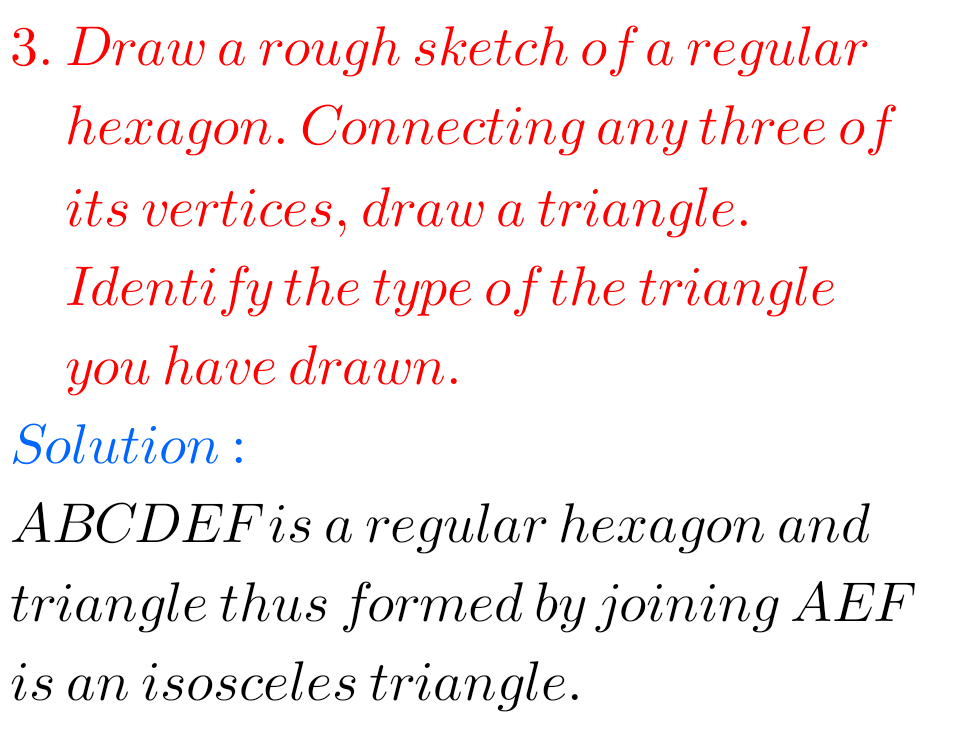FF

## Chapter 5 Understanding  Elementary Shapes Exercise 5.8 solutions Ncert class 6 Maths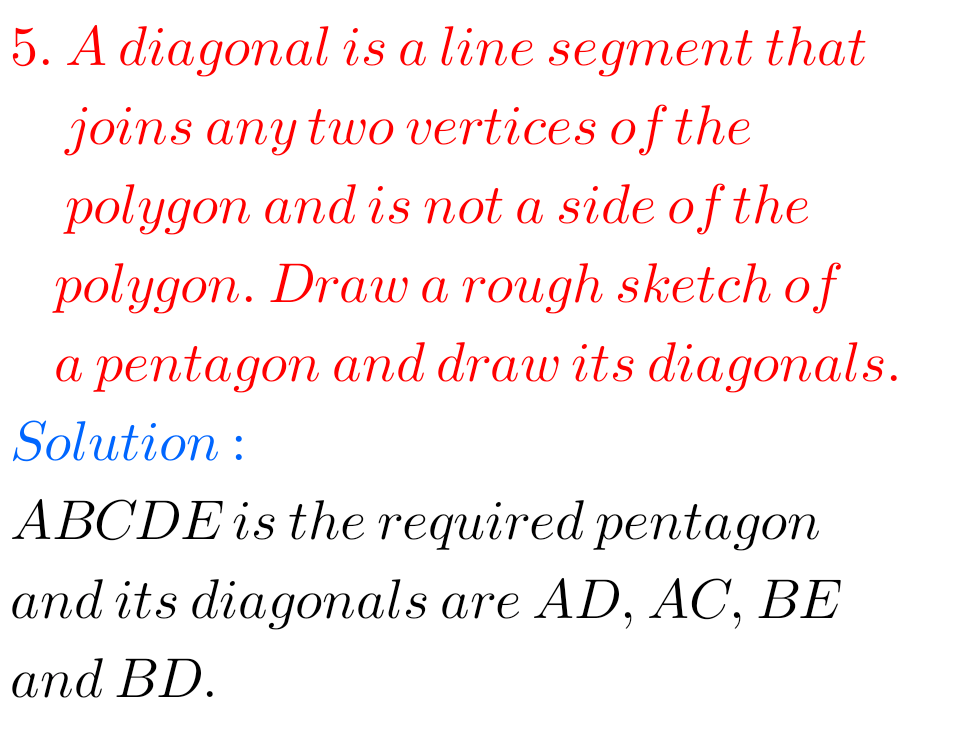F

Note : Observe the solutions and try them in your own method.